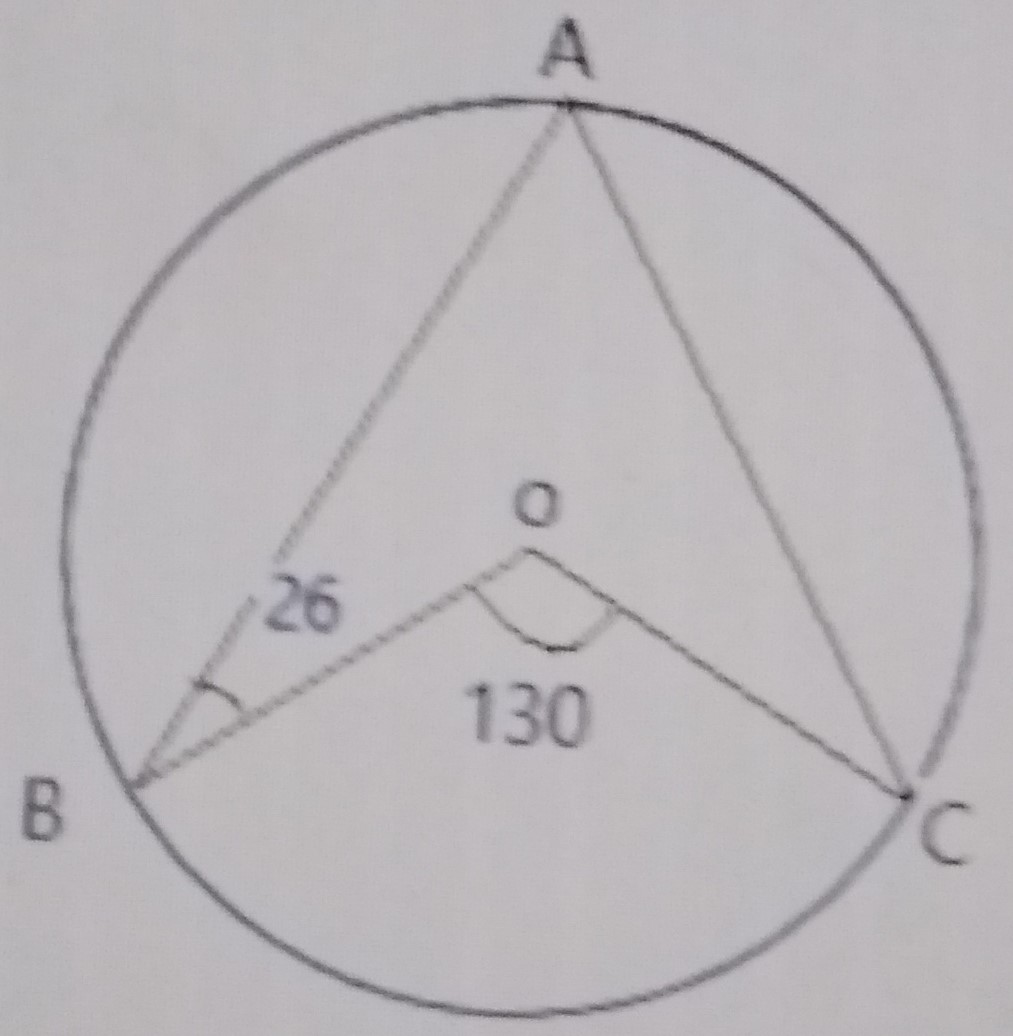# Mathematics 2019 Past Questions | JAMB

Study the following Mathematics past questions and answers for JAMBWAEC  NECO and Post-JAMB. Get prepared with official past questions and answers for upcoming examinations.

41.
Simplify $$\frac{0.0839 \times 6.381}{5.44}$$ to 2 significant figures.

• A. 0.2809
• B. 2.51
• C. 3.5
• D. 0.098
•

42. Find the value of x and y in the simultaneous equation: 3x + y = 21; xy = 30

• A. x = 3 or 7, y = 12 or 8
• B. x = 6 or 1, y = 11 or 5
• C. x = 2 or 5, y = 15 or 6
• D. x = 1 or 5, y = 10 or 7

43. Points X and Y are 20km North and 9km East of point O, respectively. What is the bearing of Y from X? Correct to the nearest degree.

• A. 24°
• B. 56°
• C. 127°
• D. 156°
44. If $$P = (\frac{Q(R – T)}{15})^{\frac{1}{3}}$$, make T the subject of the formula.

• A. $$T = \frac{15R – Q}{P^3}$$
• B. $$T = R – \frac{15P^3}{Q}$$
• C. $$T = \frac{R – 15P^3}{Q}$$
• D. $$T = \frac{R + P^3}{15Q}$$

45.In the diagram above, O is the centre of the circle ABC, < ABO = 26° and < BOC = 130°. Calculate < AOC.

• A. 26°
• B. 13°
• C. 80°
• D. 102°

46. Each of the interior angles of a regular polygon is 140°. Calculate the sum of all the interior angles of the polygon.

• A. 1080°
• B. 1260°
• C. 2160°
• D. 1800°

47. A man bought a car newly for ₦1,250,000. He had a crash with the car and later sold it at the rate of ₦1,085,000. What is the percentage gain or loss of the man?

• A. 43.7% loss
• B. 13.2% gain
• C. 13.2% loss
• D. 43.7% gain

48. If the volume of a frustrum is given as $$V = \frac{\pi h}{3} (R^2 + Rr + r^2)$$, find $$\frac{\mathrm d V}{\mathrm d R}$$.

• A. $$\frac{\pi h}{3} (2R + r)$$
• B. $$2R + r + \frac{\pi h}{3}$$
• C. $$\frac{\pi h}{3} (2R^2 + r + 2r)$$
• D. $$\frac{2R^2}{3} \pi h$$

49.  Express $$(0.0439 \div 3.62)$$ as a fraction.

• A.$$\frac{21}{100}$$
• B.$$\frac{21}{1000}$$
• C.$$\frac{12}{1000}$$
• D.$$\frac{12}{100}$$

50. If $$25^{1 – x} \times 5^{x + 2} \div (\frac{1}{125})^{x} = 625^{-1}$$, find the value of x.

• A. x = -4
• B. x = 2
• C. x = -2
• D. x = 4
Scroll to Top